# Rtu ch-2-qauntum mech

13. Jun 2021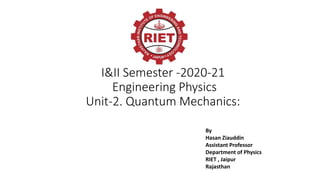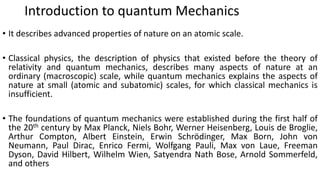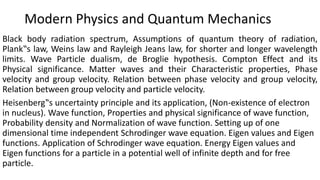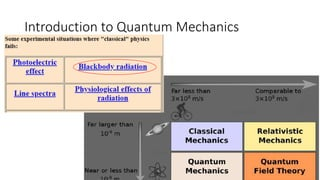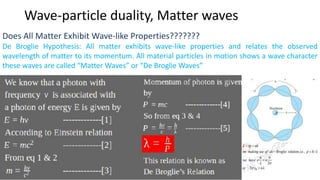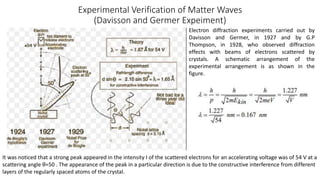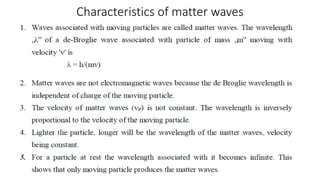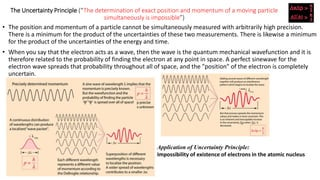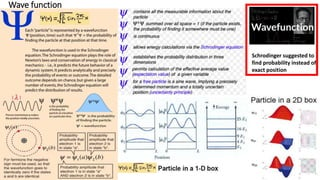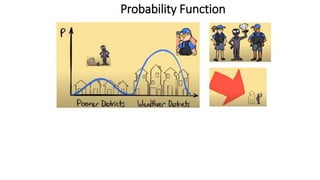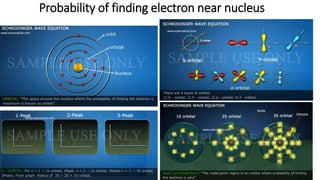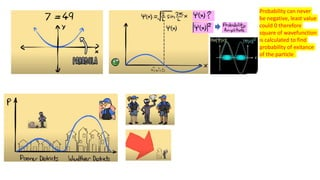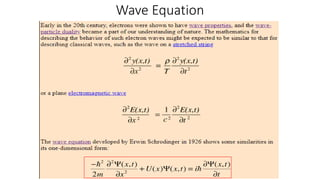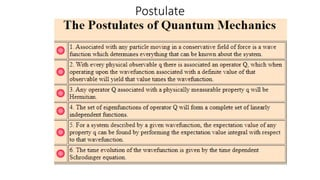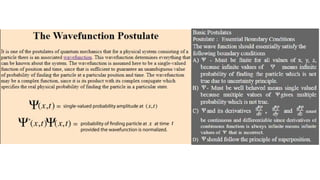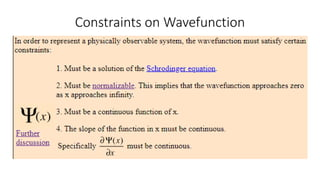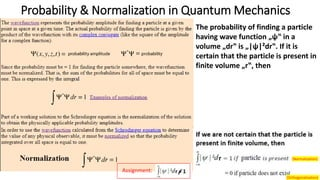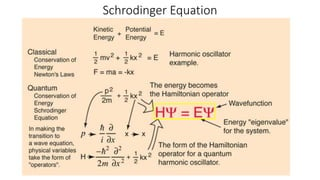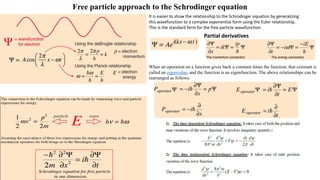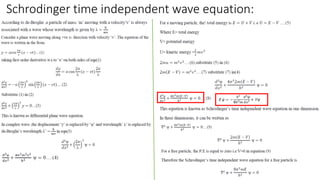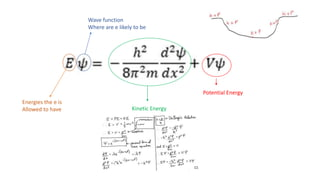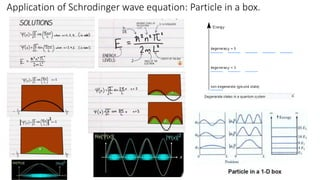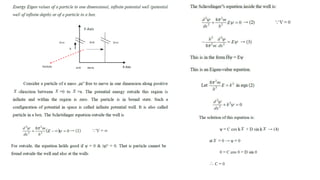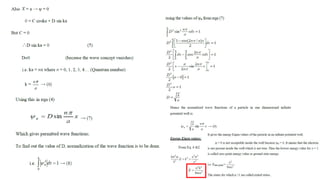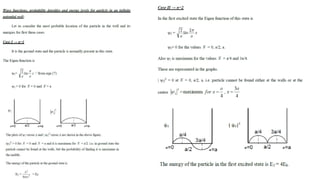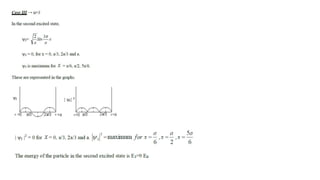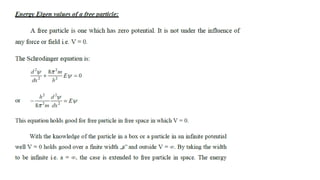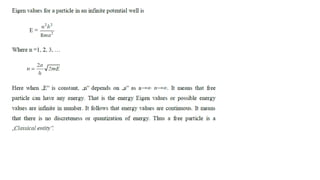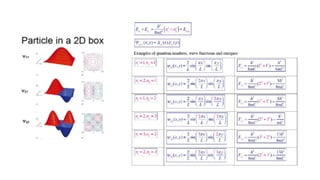1 von 29

### Rtu ch-2-qauntum mech

• 1. I&II Semester -2020-21 Engineering Physics Unit-2. Quantum Mechanics: By Hasan Ziauddin Assistant Professor Department of Physics RIET , Jaipur Rajasthan
• 2. Introduction to quantum Mechanics • It describes advanced properties of nature on an atomic scale. • Classical physics, the description of physics that existed before the theory of relativity and quantum mechanics, describes many aspects of nature at an ordinary (macroscopic) scale, while quantum mechanics explains the aspects of nature at small (atomic and subatomic) scales, for which classical mechanics is insufficient. • The foundations of quantum mechanics were established during the first half of the 20th century by Max Planck, Niels Bohr, Werner Heisenberg, Louis de Broglie, Arthur Compton, Albert Einstein, Erwin Schrödinger, Max Born, John von Neumann, Paul Dirac, Enrico Fermi, Wolfgang Pauli, Max von Laue, Freeman Dyson, David Hilbert, Wilhelm Wien, Satyendra Nath Bose, Arnold Sommerfeld, and others
• 3. Modern Physics and Quantum Mechanics Black body radiation spectrum, Assumptions of quantum theory of radiation, Plank‟s law, Weins law and Rayleigh Jeans law, for shorter and longer wavelength limits. Wave Particle dualism, de Broglie hypothesis. Compton Effect and its Physical significance. Matter waves and their Characteristic properties, Phase velocity and group velocity. Relation between phase velocity and group velocity, Relation between group velocity and particle velocity. Heisenberg‟s uncertainty principle and its application, (Non-existence of electron in nucleus). Wave function, Properties and physical significance of wave function, Probability density and Normalization of wave function. Setting up of one dimensional time independent Schrodinger wave equation. Eigen values and Eigen functions. Application of Schrodinger wave equation. Energy Eigen values and Eigen functions for a particle in a potential well of infinite depth and for free particle.
• 5. Wave-particle duality, Matter waves Does All Matter Exhibit Wave-like Properties??????? De Broglie Hypothesis: All matter exhibits wave-like properties and relates the observed wavelength of matter to its momentum. All material particles in motion shows a wave character these waves are called “Matter Waves” or “De Broglie Waves”
• 6. Experimental Verification of Matter Waves (Davisson and Germer Expeiment) It was noticed that a strong peak appeared in the intensity I of the scattered electrons for an accelerating voltage was of 54 V at a scattering angle θ=50 . The appearance of the peak in a particular direction is due to the constructive interference from different layers of the regularly spaced atoms of the crystal. Electron diffraction experiments carried out by Davisson and Germer, in 1927 and by G.P Thompson, in 1928, who observed diffraction effects with beams of electrons scattered by crystals. A schematic arrangement of the experimental arrangement is as shown in the figure.
• 8. The Uncertainty Principle (“The determination of exact position and momentum of a moving particle simultaneously is impossible”) • The position and momentum of a particle cannot be simultaneously measured with arbitrarily high precision. There is a minimum for the product of the uncertainties of these two measurements. There is likewise a minimum for the product of the uncertainties of the energy and time. • When you say that the electron acts as a wave, then the wave is the quantum mechanical wavefunction and it is therefore related to the probability of finding the electron at any point in space. A perfect sinewave for the electron wave spreads that probability throughout all of space, and the "position" of the electron is completely uncertain. Application of Uncertainty Principle: Impossibility of existence of electrons in the atomic nucleus
• 9. Wave function Schrodinger suggested to find probability instead of exact position
• 11. Probability of finding electron near nucleus
• 12. Probability can never be negative, least value could 0 therefore square of wavefunction is calculated to find probability of exitance of the particle
• 17. Probability & Normalization in Quantum Mechanics The probability of finding a particle having wave function „ψ‟ in a volume „dr‟ is „|ψ|²dr‟. If it is certain that the particle is present in finite volume „r‟, then Assignment: (Orthogonalization) (Normalization)
• 19. Free particle approach to the Schrodinger equation It is easier to show the relationship to the Schrodinger equation by generalizing this wavefunction to a complex exponential form using the Euler relationship. This is the standard form for the free particle wavefunction. Partial derivatives When an operation on a function gives back a constant times the function, that constant is called an eigenvalue, and the function is an eigenfunction. The above relationships can be rearranged as follows.
• 21. Wave function Where are e likely to be Energies the e is Allowed to have Potential Energy Kinetic Energy
• 22. Application of Schrodinger wave equation: Particle in a box.